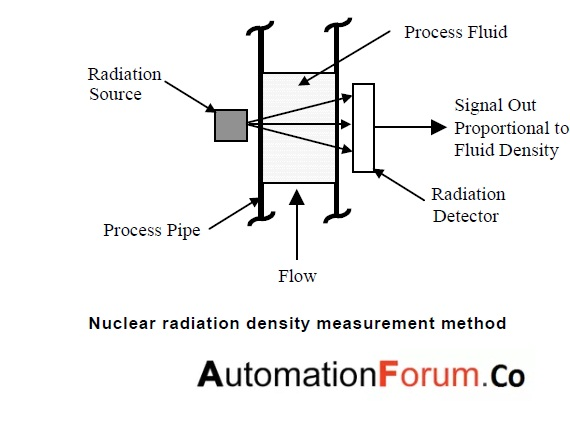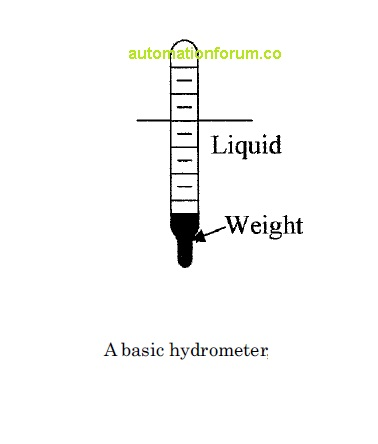# density## Measuring density using nuclear radiation

Introduction There are so many instruments to measure density like hydrometers,Induction hydrometers,etc You can also use nuclear devices to measure density.In this session we are going to discuss about the nuclear devices to measure density Working principle   They operate on the principle that the absorption of gamma radiation increases with the density of the …## Density measuring devices

Introduction In this session we are going to discuss about density measuring devices ,before that we need to go through the basics. Density ρ  of a material is defined as the mass per unit volume. Units of density are pounds (slug) per cubic foot [lb (slug)/ft3] or kilogram per cubic meter (kg/m3). Specific weight γ is defined …

Scroll to Top